#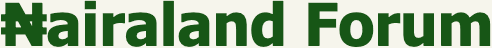Welcome, Guest: Join Nairaland / LOGIN! / Trending / Recent / New
Stats: 2,624,417 members, 6,118,976 topics. Date: Tuesday, 26 January 2021 at 03:02 AM

## How To Calculate The Quantity Of Tiles Needed; Selecting Tiles & Types. - Properties - Nairaland

(1) (2) (3) (4) (Reply) (Go Down)

 How To Calculate The Quantity Of Tiles Needed; Selecting Tiles & Types. by saydfact(m): 1:27pm On Sep 16, 2019 GoodDay and its good to be back.Let's go practical (as we always do) - calculating the number of tiles needed to tile various spaces within the building (floor and wall tiling).We would make reference to our 3 bedroom plan, designed specifically for Nairaland lectures and calculate the tiles needed in the living room, one of the bedrooms and the master's toilet's floor and wall tiles.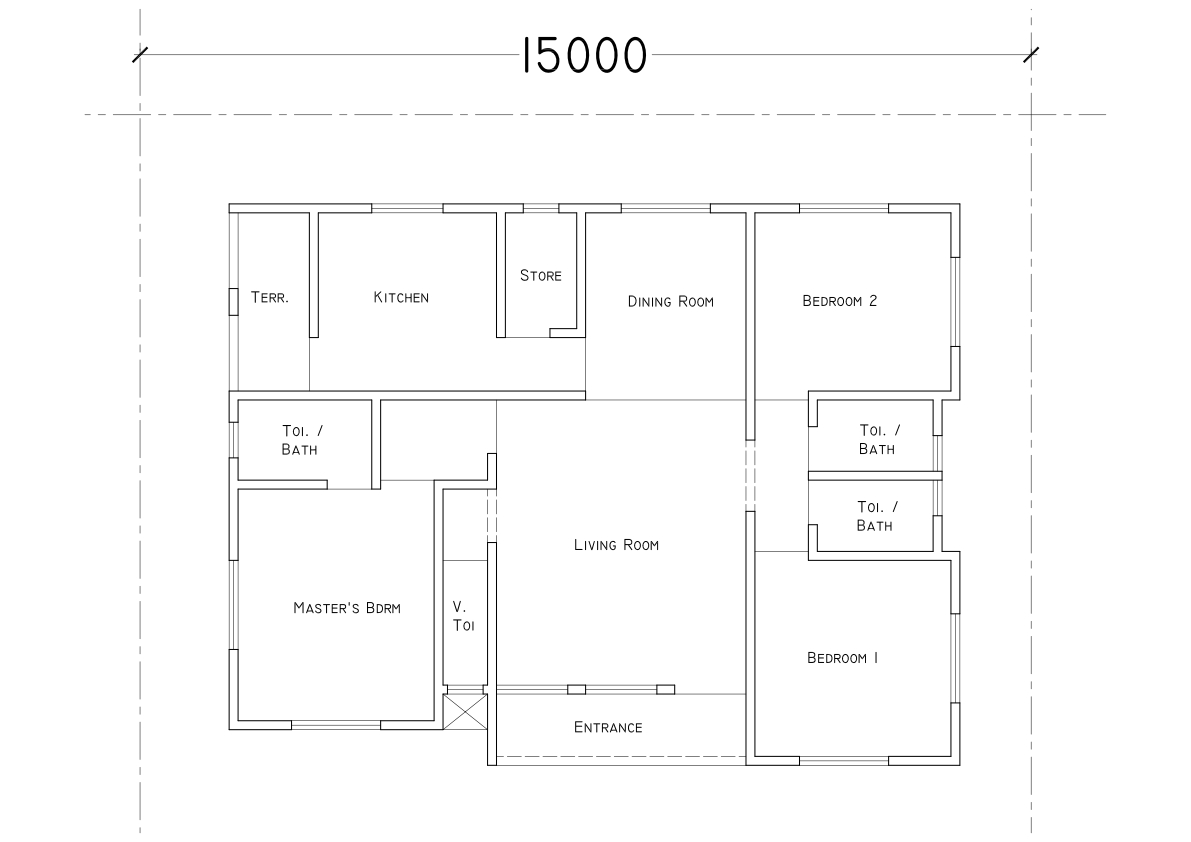SELECTING TILES1. Tiles come in various sizes, the common ones being 150x150, 200x200, 300x300, 150x300, 200x400, 300x600, 400x400 and 600x600; the first thing to do is pick a tile (based on clients choice or use) for each floor and wall. There is no rule stopping you from using any particular tile size for floor or wall, as long as it has the perfect surface finish for that use.e.g, a glazed (slippery) tile is not appropriate in the bathroom, so if I find any non-slippery tile, irrespective of the size, I can use it for my wet floors. Wet floors include Bathroom, Swimming pool, Laundry, etc. (We've had buildings where 600x600 tiles were used on the wall and 150x150 tiles used on the floor) - in summary, the tile finish NOT the tile size determines where it is used.2. Select tiles for each space. (Let's select ours too).I'll go with the popular opinion; Living Room (600x600), Bedroom (400x400), Bathroom Floor (300x300) and Bathroom Wall (200x400).Note:* Glazed tiles cannot be used on wet floors - it's very slippery if the floor is wet and dangerous* Un-Glazed, Matte, Rough tiles can be used on any surface (wet or dry)3. Calculate the area of the spaces (floor and wall) separately - This uses the simple LxB (area of a rectangle principle) - and write it out.Note:* Remember to deduct door and window openings where applicable (e.g Area of wall = 10m2; area of window = 0.5m2 therefore area to be tiled = 10m2 - 0.5m2 = 9.5m2)* After getting your area to be tiled, ADD 10% of quantity for waste & broken tiles. (e.g 9.5m2 + 10% of 9.5m2 >> 9.5 + 0.95 >> 10.45m2)4. Calculate the Total area of Tiles you're picking; this varies from one manufacturer to the other and from one product size to the other. >> this means that while 400x400 tiles from SaydFact Ceramics contain 11 pcs, those of Teewhy2 Tiles Ltd might contain 13 pcs - It is, therefore, important to know how much pieces each pack of tiles you're buying contain.5. Ascertain the total area of one pack of tiles (either by calculating or checking on the pack), use this figure to divide the total square area of the room to be tiled and the result gives you quantities needed - in simple terms >> (AREA OF LIVING ROOM / area of 1 pack of tiles) = Quantity of Tiles needed (remember to add 10% for waste).LETS NOW GO PRACTICAL BY CALCULATING USING THE ATTACHED DRAWING (see pics below) and information in No2 above.A. Master's Bedroom:* From the diamond below; total floor area of Master's Rm is 13.20m2* Lets agree that our 400x400 tiles contain 11 pieces; hence area of 1 pack in meters >> 0.4m x 0.4m x 11 pcs (400mm is same as 0.4m and area of 1 tile is 0.4 x 0.4, while the area of 1 pack is (Area of 1 tile x number of tiles in 1 pack)1 PACK = 0.4 x 0.4 x 11 = 1.76m2Number of tiles needed to complete Master's Rm floor = 13.20 (divided by) 1.76m2 = 13.20 / 1.76 = 7.5 packsbefore we add for waste; let's calculate skirting for the Bedroom using 120mm as skirting height.* The 1st question is how many skirting can we get from 1 tile? Ans: Lenght of tiles / Height of skirting = 400 / 120 = 3 skirting from 1 tiles.* What is the perimeter of the space needing skirting?Ans: From the diagram, bedroom is 4150 by 3150 hence perimeter of room = 4150 + 4150 + 3150 + 3150 = 14600mm * Deduct openings from the perimeter ie 2 doors (toilet and bedroom doors) where there won't be need for skirting >> 750+900 = 1650mm* Length requiring skirting = 14600mm - 1650mm = 12950mm* Numbers of Skirting needed = 12950 / 400 (lenght of 1 tile) = 33 pcs (approximate to the next whole nos)* Number of Tiles needed to make requirement = 33 pcs / 3 (number of skirting gotten from 1 tile) = 11 pcs (11 tiles are needed for skirting)* Number of packs needed for skirting = 11 pcs / 11 pcs (number in 1 pack) = 1 pack is needed.TOTAL TILES NEEDED FOR MASTER'S BEDROOM:7.5 PACKS (FLOOR) + 1 PACK (SKIRTING) = 8.5 PACKS.ADD 10% FOR WASTE >> 8.5 Packs + 10% of 8.5 = 8.5+ 0.85 = 9.35 packs neededHENCE BUY 10 PACKS (approximated to the next whole number because you can't buy half tiles)B. Living Room: (Straight to Biz)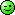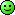We're using 600x600 tiles and each pack contains 4 pcs; hence Area of 1 pack = 0.6x0.6x4 = 1.44m2Area of Living Room (see diagram) = 22.02m2* Number of Packs needed for Floor >> 22.02 / 1.44 = 15.2 packsSkirting >> Perimeter of Living Room - (All Openings) = 12.2m or 12200mm* Number of Skirting in 1 tiles >> 600 / 120 = 5 pcs (from 1 tiles)* Number of skirting needed for Living Room = 12200 / 600 = 21 pcs* Number of Tiles needed to meet requirements = 21 / 5 = 5 tiles* Number of packs needed for skirting = 5 tiles / 4 pcs (number in 1 pack) = 1.25 pack is needed.TOTAL TILES NEEDED FOR LIVING ROOM:15.2 PACKS (FLOOR) + 1.25 PACK (SKIRTING) = 17 PACKS.ADD 10% FOR WASTE >> 17 Packs + 10% of 17 = 17+ 1.7 = 18.7 packs neededHENCE BUY 19 PACKS (approximated to the next whole number because you can't buy half tiles)C. Bathroom Floor: (using 300mm by 300mm Tiles)Area of Floor / Area of 1 pack of Tiles >> 3.26 / 1.35 (assuming nos in 1 pack = 15nos) = 2.4 packsThere's no skirting in Bathroom because we have wall tiles.TOTAL TILES NEEDED FOR BATHROOM FLOOR = 2.4 + 10% of 2.4 = 3 PACKS D. Bathroom Wall:We're using 200x400 tiles and each pack contains 17 pcs; hence Area of 1 pack = 0.2x0.4x17 = 1.36m2Let's also assume that we're tiling up-to 2.1m only i.e Window level (the alternative is to tile up-to 3m; i.e ceiling level)* What is the perimeter of the space needing wall tiles?Ans: From the diagram, bathroom is 2100 by 1500 hence perimeter of room = 2100 + 2100 + 1500 + 1500 = 7200mm = 7.2m* Find total area to be tiled >> Perimeter x Height used >> 7.2m x 2.1m = 15.12m2* Deduct Area of openings from the Area above i.e 1 door (2.1m x 0.75m) and 1 window (0.6m x 0.6m) >> (1.6m2 + 0.36m2) = 1.96m2* Hence; Area to be tiled = 15.12m2 - 1.96m2 = 13.16m2Now we revert to our earlier formulas i.e AREAS TO BE TILED / AREA OF 1 PACK = NUMBERS OF PACKS NEEDED* 13.16m2 / 1.36m2 (area of 1 pack) = 9.7 packs = 10 packs (approximate to next whole number)TOTAL TILES NEEDED FOR TOILET WALL: 10 PACKSADD 10% FOR WASTE >> 10 Packs + 10% of 10 = 10 + 1 = 11 packs neededHENCE BUY 11 PACKS Do the above for all space in the building; You can even prepare an excel file with the formulas so you won't need calculations each time; just input the area of floor and area of tiles and bingo.Thanks for Following... 61 Likes 26 Shares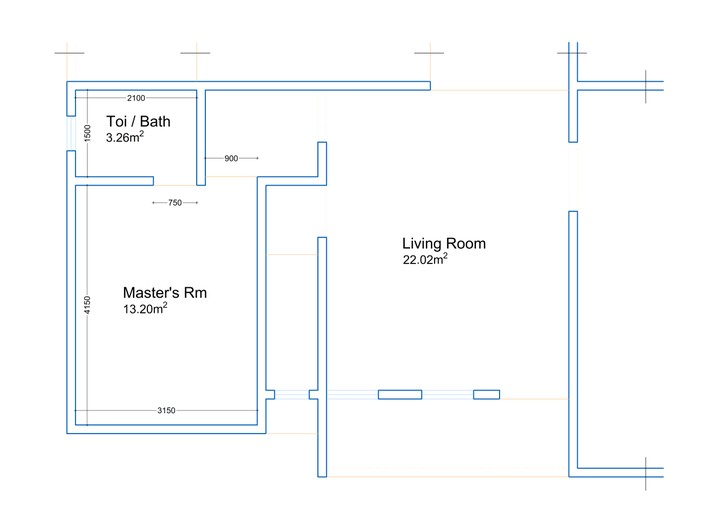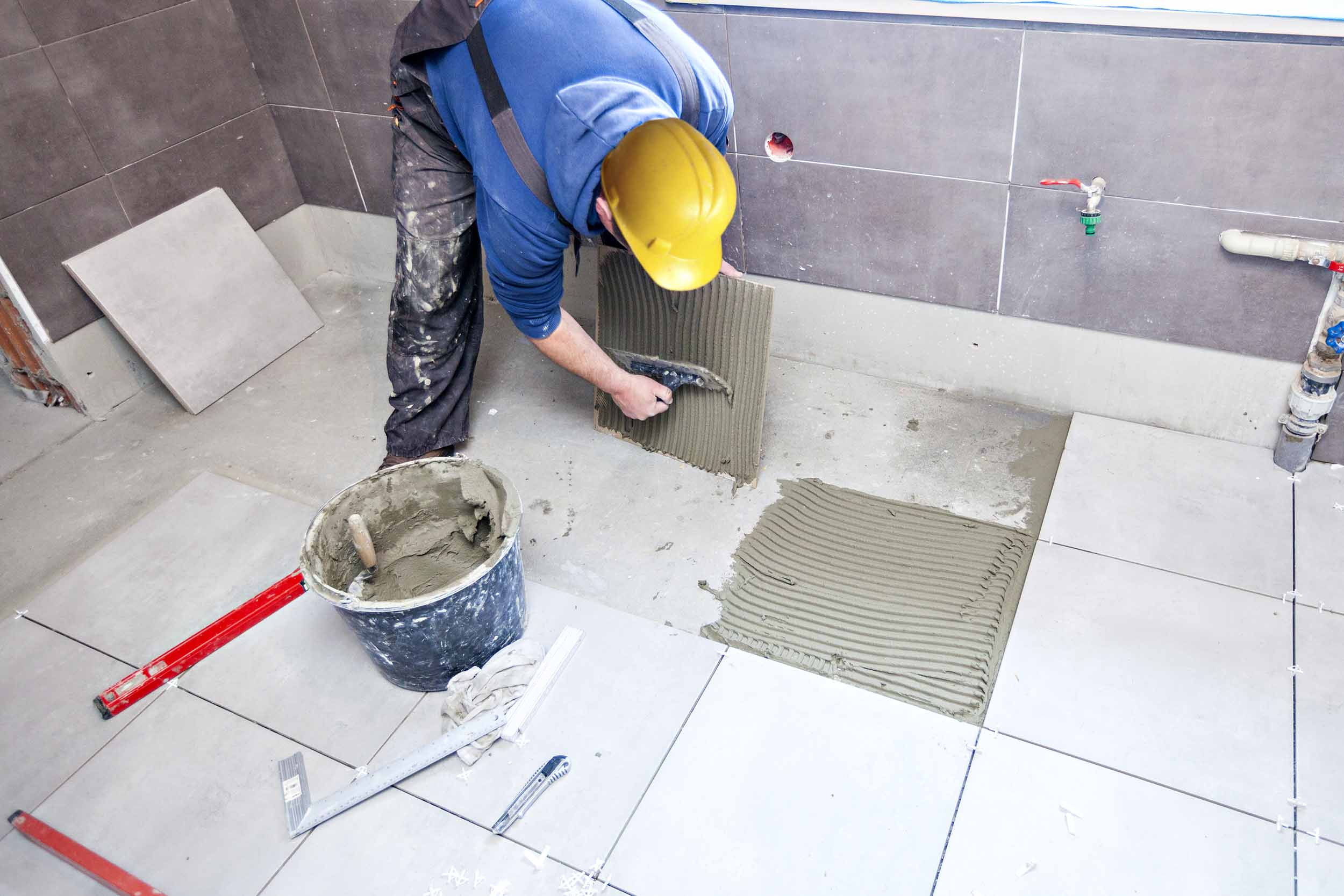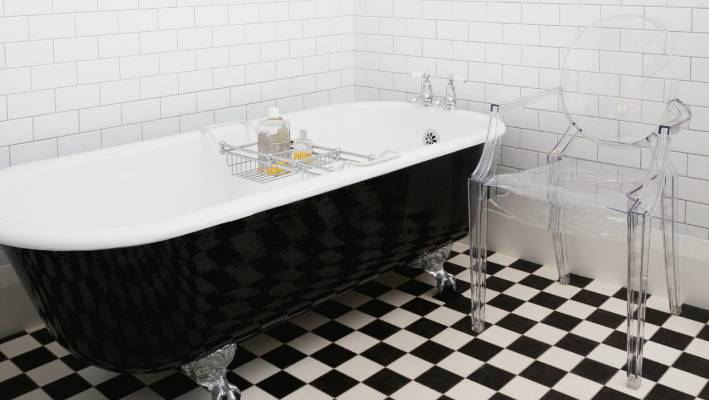Re: How To Calculate The Quantity Of Tiles Needed; Selecting Tiles & Types. by shamsin6300(m): 4:11pm On Sep 16, 2019 The guy aboveY do u snatch my FCT 1 Like Re: How To Calculate The Quantity Of Tiles Needed; Selecting Tiles & Types. by popsyleo1: 4:11pm On Sep 16, 2019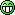Re: How To Calculate The Quantity Of Tiles Needed; Selecting Tiles & Types. by fajob: 4:12pm On Sep 16, 2019 Wow 1 Like Re: How To Calculate The Quantity Of Tiles Needed; Selecting Tiles & Types. by Acehart: 4:12pm On Sep 16, 2019 Very educative 1 Like 1 Share Re: How To Calculate The Quantity Of Tiles Needed; Selecting Tiles & Types. by xcelentattitude(m): 4:13pm On Sep 16, 2019 . 5 Likes 1 ShareRe: How To Calculate The Quantity Of Tiles Needed; Selecting Tiles & Types. by Sirpaul(m): 4:13pm On Sep 16, 2019 na your network do you strong thing shamsin6300:The guy aboveY do u snatch my FCT Re: How To Calculate The Quantity Of Tiles Needed; Selecting Tiles & Types. by holina: 4:13pm On Sep 16, 2019 op . u taking food from quantity surveyors mouth Re: How To Calculate The Quantity Of Tiles Needed; Selecting Tiles & Types. by Siralgebra04(m): 4:13pm On Sep 16, 2019 Let me go and carry my giant calculator.In d meantime, I have a brief analysis here. If u burn #2,145 (15litres) fuel, say, every 3 days, then, in a year you would have expended # 260,975 fuel + #20,000 for maintenance. In 2 years u would av spent over 560k. That's too massive.Embrace Solar Energy. At Medas Innovations, we will be willing to mount ur Solar System.Check my signature for contact. 2 Likes Re: How To Calculate The Quantity Of Tiles Needed; Selecting Tiles & Types. by Nobody: 4:13pm On Sep 16, 2019 Amazing calculations.How many pcs of tiles do we need for the whole nairaland website.15 Likes 2 SharesRe: How To Calculate The Quantity Of Tiles Needed; Selecting Tiles & Types. by Nwaohafia1: 4:13pm On Sep 16, 2019 Good info, but Why disturb my brain when I have an engineer that I am paying for the job? 11 Likes Re: How To Calculate The Quantity Of Tiles Needed; Selecting Tiles & Types. by Puregold3(f): 4:13pm On Sep 16, 2019 Awesome info.. how do I also calculate the amount of cement needed to complete a building? 7 Likes 1 Share Re: How To Calculate The Quantity Of Tiles Needed; Selecting Tiles & Types. by lizdammy: 4:14pm On Sep 16, 2019 Nice 1 Like Re: How To Calculate The Quantity Of Tiles Needed; Selecting Tiles & Types. by Sirseedorf(m): 4:14pm On Sep 16, 2019 Nice one But next time don't write epistle thinking am going to read it all.Anyways either full or broken tile, it's still usable.. Re: How To Calculate The Quantity Of Tiles Needed; Selecting Tiles & Types. by Crazeworld(m): 4:14pm On Sep 16, 2019 Bookmarked Meanwhile, I'm available for your online documents and Print-out Slips. Check my signature Re: How To Calculate The Quantity Of Tiles Needed; Selecting Tiles & Types. by Nobody: 4:14pm On Sep 16, 2019 What about screeding.shouldnt you calculate that as well since it forms part of the cost of tiles? And your metal wetin call for the corners and edges? And your white or black cement?To avoid variation matterWell done Re: How To Calculate The Quantity Of Tiles Needed; Selecting Tiles & Types. by makavelli80(m): 4:15pm On Sep 16, 2019 So it must always be a white man when it comes to advert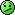2 Likes Re: How To Calculate The Quantity Of Tiles Needed; Selecting Tiles & Types. by xcelentattitude(m): 4:15pm On Sep 16, 2019 ... 3 LikesRe: How To Calculate The Quantity Of Tiles Needed; Selecting Tiles & Types. by dejonathan(m): 4:15pm On Sep 16, 2019 This trend is not suppose to be on front page...How do you expect we wey dey do the work to dey see extra small small change from tiles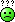Re: How To Calculate The Quantity Of Tiles Needed; Selecting Tiles & Types. by miteolu(m): 4:15pm On Sep 16, 2019 THANKS 1 ShareRe: How To Calculate The Quantity Of Tiles Needed; Selecting Tiles & Types. by IronPant(m): 4:15pm On Sep 16, 2019 Op 90% of nairalanders won't like thisThey only want Bullsh!t naija and yeyebrities topping front page 3 Likes 1 ShareRe: How To Calculate The Quantity Of Tiles Needed; Selecting Tiles & Types. by metroid(m): 4:16pm On Sep 16, 2019 Thanks OP 7 Likes 1 ShareRe: How To Calculate The Quantity Of Tiles Needed; Selecting Tiles & Types. by dejonathan(m): 4:17pm On Sep 16, 2019 holina:op . u taking food from quantity surveyors mouthGBAM Re: How To Calculate The Quantity Of Tiles Needed; Selecting Tiles & Types. by ZombieTAMER: 4:17pm On Sep 16, 2019 Nice one Re: How To Calculate The Quantity Of Tiles Needed; Selecting Tiles & Types. by BlessedNuel(m): 4:19pm On Sep 16, 2019 Nice one....kudos to the OP. Meanwhile if you need Pure Original Honey... Kindly call or whatsapp me on the number attached to my signature below Re: How To Calculate The Quantity Of Tiles Needed; Selecting Tiles & Types. by RockOfAgesAlumi(m): 4:19pm On Sep 16, 2019 Good afternoon all! Re: How To Calculate The Quantity Of Tiles Needed; Selecting Tiles & Types. by dasoul(m): 4:19pm On Sep 16, 2019 Thanks for sharing bro. It was really informative. We need more of this on nairaland. 1 Like 1 Share Re: How To Calculate The Quantity Of Tiles Needed; Selecting Tiles & Types. by Mcsammy4u(m): 4:20pm On Sep 16, 2019 Very informativesaydfact:GoodDay and its good to be back.Let's go practical (as we always do) - calculating the number of tiles needed to tile various spaces within the building (floor and wall tiling).We would make reference to our 3 bedroom plan, designed specifically for Nairaland lectures and calculate the tiles needed in the living room, one of the bedrooms and the master's toilet's floor and wall tiles.SELECTING TILES1. Tiles come in various sizes, the common ones being 150x150, 200x200, 300x300, 150x300, 200x400, 300x600, 400x400 and 600x600; the first thing to do is pick a tile (based on clients choice or use) for each floor and wall. There is no rule stopping you from using any particular tile size for floor or wall, as long as it has the perfect surface finish for that use.e.g, a glazed (slippery) tile is not appropriate in the bathroom, so if I find any non-slippery tile, irrespective of the size, I can use it for my wet floors. Wet floors include Bathroom, Swimming pool, Laundry, etc. (We've had buildings where 600x600 tiles were used on the wall and 150x150 tiles used on the floor) - in summary, the tile finish NOT the tile size determines where it is used.2. Select tiles for each space. (Let's select ours too).I'll go with the popular opinion; Living Room (600x600), Bedroom (400x400), Bathroom Floor (300x300) and Bathroom Wall (200x400).Note:* Glazed tiles cannot be used on wet floors - it's very slippery if the floor is wet and dangerous* Un-Glazed, Matte, Rough tiles can be used on any surface (wet or dry)3. Calculate the area of the spaces (floor and wall) separately - This uses the simple LxB (area of a rectangle principle) - and write it out.Note:* Remember to deduct door and window openings where applicable (e.g Area of wall = 10m2; area of window = 0.5m2 therefore area to be tiled = 10m2 - 0.5m2 = 9.5m2)* After getting your area to be tiled, ADD 10% of quantity for waste & broken tiles. (e.g 9.5m2 + 10% of 9.5m2 >> 9.5 + 0.95 >> 10.45m2)4. Calculate the Total area of Tiles you're picking; this varies from one manufacturer to the other and from one product size to the other. >> this means that while 400x400 tiles from SaydFact Ceramics contain 11 pcs, those of Teewhy2 Tiles Ltd might contain 13 pcs - It is, therefore, important to know how much pieces each pack of tiles you're buying contain.5. Ascertain the total area of one pack of tiles (either by calculating or checking on the pack), use this figure to divide the total square area of the room to be tiled and the result gives you quantities needed - in simple terms >> (AREA OF LIVING ROOM / area of 1 pack of tiles) = Quantity of Tiles needed (remember to add 10% for waste).LETS NOW GO PRACTICAL BY CALCULATING USING THE ATTACHED DRAWING (see pics below) and information in No2 above.A. Master's Bedroom:* From the diamond below; total floor area of Master's Rm is 13.20m2* Lets agree that our 400x400 tiles contain 11 pieces; hence area of 1 pack in meters >> 0.4m x 0.4m x 11 pcs (400mm is same as 0.4m and area of 1 tile is 0.4 x 0.4, while the area of 1 pack is (Area of 1 tile x number of tiles in 1 pack)1 PACK = 0.4 x 0.4 x 11 = 1.76m2Number of tiles needed to complete Master's Rm floor = 13.20 (divided by) 1.76m2 = 13.20 / 1.76 = 7.5 packsbefore we add for waste; let's calculate skirting for the Bedroom using 120mm as skirting height.* The 1st question is how many skirting can we get from 1 tile? Ans: Lenght of tiles / Height of skirting = 400 / 120 = 3 skirting from 1 tiles.* What is the perimeter of the space needing skirting?Ans: From the diagram, bedroom is 4150 by 3150 hence perimeter of room = 4150 + 4150 + 3150 + 3150 = 14600mm * Deduct openings from the perimeter ie 2 doors (toilet and bedroom doors) where there won't be need for skirting >> 750+900 = 1650mm* Length requiring skirting = 14600mm - 1650mm = 12950mm* Numbers of Skirting needed = 12950 / 400 (lenght of 1 tile) = 33 pcs (approximate to the next whole nos)* Number of Tiles needed to make requirement = 33 pcs / 3 (number of skirting gotten from 1 tile) = 11 pcs (11 tiles are needed for skirting)* Number of packs needed for skirting = 11 pcs / 11 pcs (number in 1 pack) = 1 pack is needed.TOTAL TILES NEEDED FOR MASTER'S BEDROOM:7.5 PACKS (FLOOR) + 1 PACK (SKIRTING) = 8.5 PACKS.ADD 10% FOR WASTE >> 8.5 Packs + 10% of 8.5 = 8.5+ 0.85 = 9.35 packs neededHENCE BUY 10 PACKS (approximated to the next whole number because you can't buy half tiles)B. Living Room: (Straight to Biz)We're using 600x600 tiles and each pack contains 4 pcs; hence Area of 1 pack = 0.6x0.6x4 = 1.44m2Area of Living Room (see diagram) = 22.02m2* Number of Packs needed for Floor >> 22.02 / 1.44 = 15.2 packsSkirting >> Perimeter of Living Room - (All Openings) = 12.2m or 12200mm* Number of Skirting in 1 tiles >> 600 / 120 = 5 pcs (from 1 tiles)* Number of skirting needed for Living Room = 12200 / 600 = 21 pcs* Number of Tiles needed to meet requirements = 21 / 5 = 5 tiles* Number of packs needed for skirting = 5 tiles / 4 pcs (number in 1 pack) = 1.25 pack is needed.TOTAL TILES NEEDED FOR LIVING ROOM:15.2 PACKS (FLOOR) + 1.25 PACK (SKIRTING) = 17 PACKS.ADD 10% FOR WASTE >> 17 Packs + 10% of 17 = 17+ 1.7 = 18.7 packs neededHENCE BUY 19 PACKS (approximated to the next whole number because you can't buy half tiles)C. Bathroom Floor: (using 300mm by 300mm Tiles)Area of Floor / Area of 1 pack of Tiles >> 3.26 / 1.35 (assuming nos in 1 pack = 15nos) = 2.4 packsThere's no skirting in Bathroom because we have wall tiles.TOTAL TILES NEEDED FOR BATHROOM FLOOR = 2.4 + 10% of 2.4 = 3 PACKS D. Bathroom Wall:We're using 200x400 tiles and each pack contains 17 pcs; hence Area of 1 pack = 0.2x0.4x17 = 1.36m2Let's also assume that we're tiling up-to 2.1m only i.e Window level (the alternative is to tile up-to 3m; i.e ceiling level)* What is the perimeter of the space needing wall tiles?Ans: From the diagram, bathroom is 2100 by 1500 hence perimeter of room = 2100 + 2100 + 1500 + 1500 = 7200mm = 7.2m* Find total area to be tiled >> Perimeter x Height used >> 7.2m x 2.1m = 15.12m2* Deduct Area of openings from the Area above i.e 1 door (2.1m x 0.75m) and 1 window (0.6m x 0.6m) >> (1.6m2 + 0.36m2) = 1.96m2* Hence; Area to be tiled = 15.12m2 - 1.96m2 = 13.16m2Now we revert to our earlier formulas i.e AREAS TO BE TILED / AREA OF 1 PACK = NUMBERS OF PACKS NEEDED* 13.16m2 / 1.36m2 (area of 1 pack) = 9.7 packs = 10 packs (approximate to next whole number)TOTAL TILES NEEDED FOR TOILET WALL: 10 PACKSADD 10% FOR WASTE >> 10 Packs + 10% of 10 = 10 + 1 = 11 packs neededHENCE BUY 11 PACKS Do the above for all space in the building; You can even prepare an excel file with the formulas so you won't need calculations each time; just input the area of floor and area of tiles and bingo.Thanks for Following... 4 Likes 1 Share Re: How To Calculate The Quantity Of Tiles Needed; Selecting Tiles & Types. by dwas: 4:21pm On Sep 16, 2019 Nice one 1 Like 1 Share Re: How To Calculate The Quantity Of Tiles Needed; Selecting Tiles & Types. by Callertunez: 4:24pm On Sep 16, 2019 Amuatulu:Amazing calculations. How many pcs of tiles do we need for the whole nairaland website.1 Like Re: How To Calculate The Quantity Of Tiles Needed; Selecting Tiles & Types. by Nobody: 4:26pm On Sep 16, 2019 Nwaohafia1:Good info, but Why disturb my brain when I have an engineer that I am paying for the job? So that you are not cheated.no be naija we dey? 4 Likes 2 Shares